Скачать презентацию Physics of Semiconductor Devices Lecture 5. The

lecture_5.pptx

• Размер: 1.1 Мб
• Автор:
• Количество слайдов: 33

## Описание презентации Physics of Semiconductor Devices Lecture 5. The по слайдам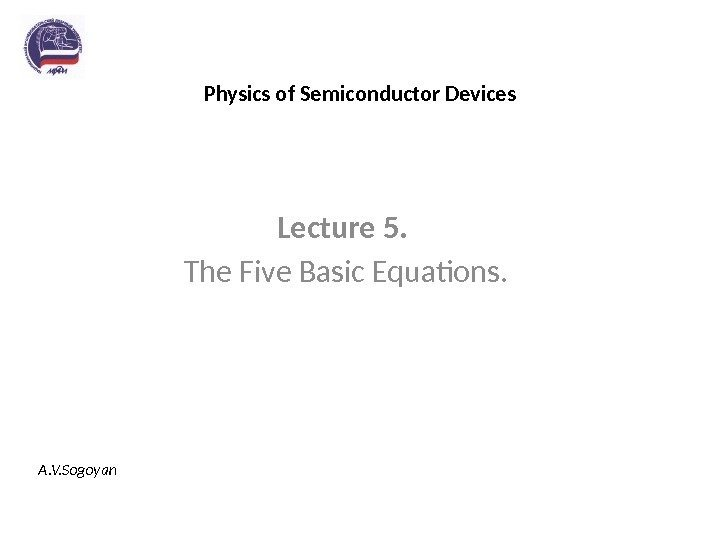Physics of Semiconductor Devices Lecture 5. The Five Basic Equations. A. V. Sogoyan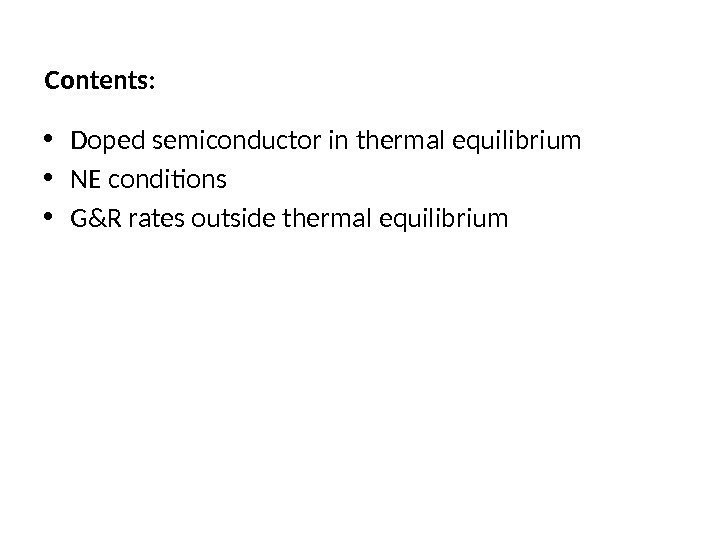Contents: • Doped semiconductor in thermal equilibrium • NE conditions • G&R rates outside thermal equilibrium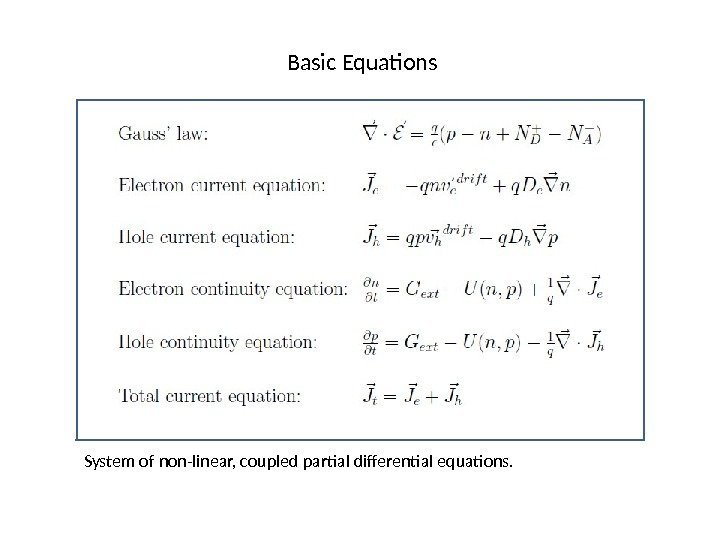Basic Equations System of non-linear, coupled partial differential equations.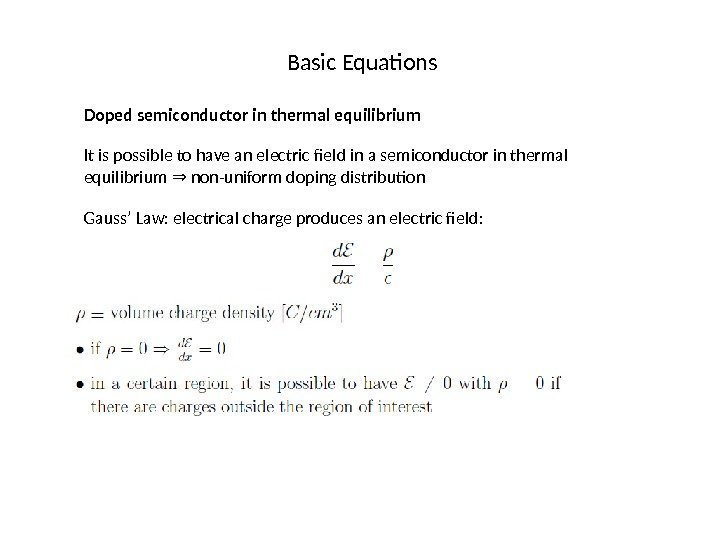Basic Equations Doped semiconductor in thermal equilibrium It is possible to have an electric field in a semiconductor in thermal equilibrium non-uniform doping distribution⇒ Gauss’ Law: electrical charge produces an electric field: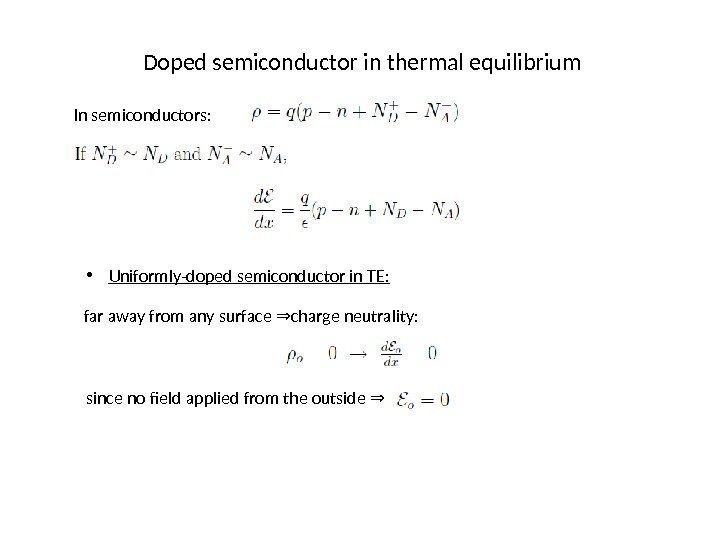Doped semiconductor in thermal equilibrium In semiconductors: • Uniformly-doped semiconductor in TE: far away from any surface charge neutrality: ⇒ since no field applied from the outside ⇒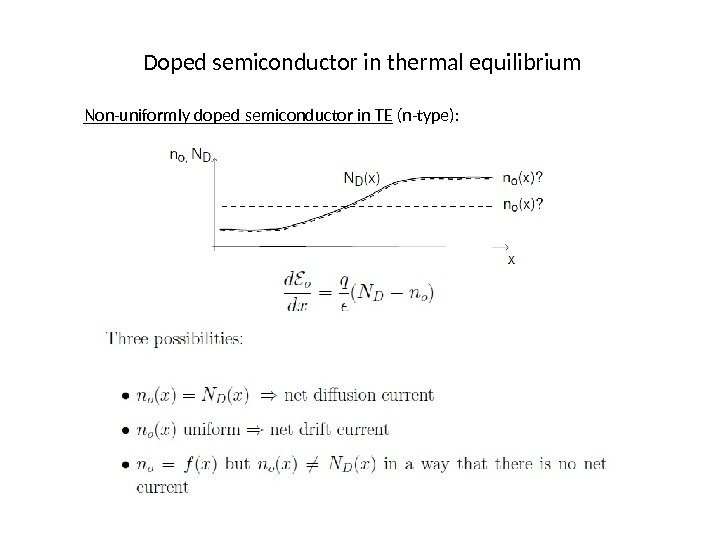Doped semiconductor in thermal equilibrium Non-uniformly doped semiconductor in TE (n-type):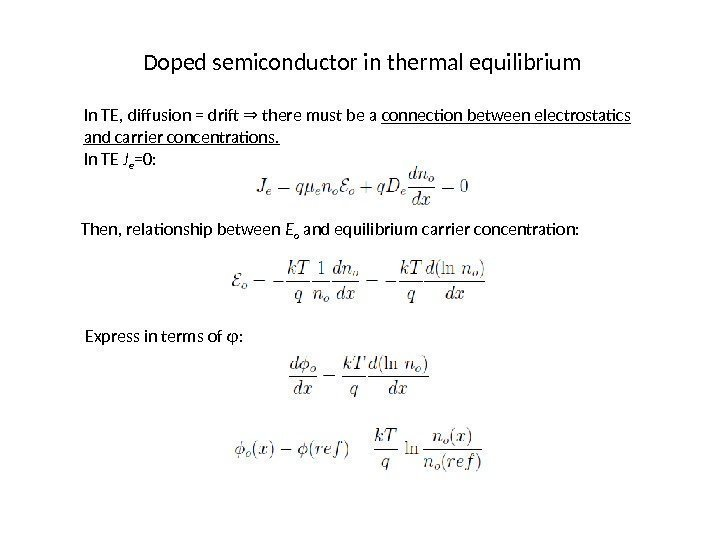Doped semiconductor in thermal equilibrium In TE, diffusion = drif there must be a ⇒ connection between electrostatics and carrier concentrations. In TE J e =0: Then, relationship between E o and equilibrium carrier concentration: Express in terms of φ: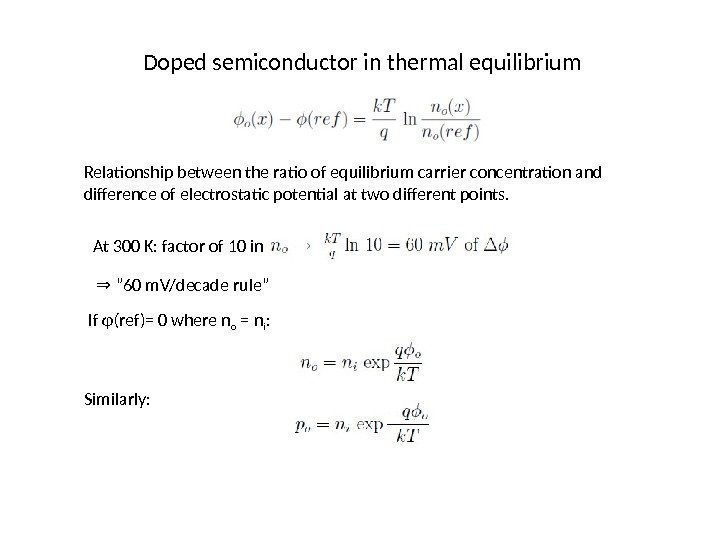Doped semiconductor in thermal equilibrium Relationship between the ratio of equilibrium carrier concentration and difference of electrostatic potential at two different points. At 300 K: factor of 10 in ”⇒ 60 m. V/decade rule” If φ(ref)= 0 where n o = n i : Similarly: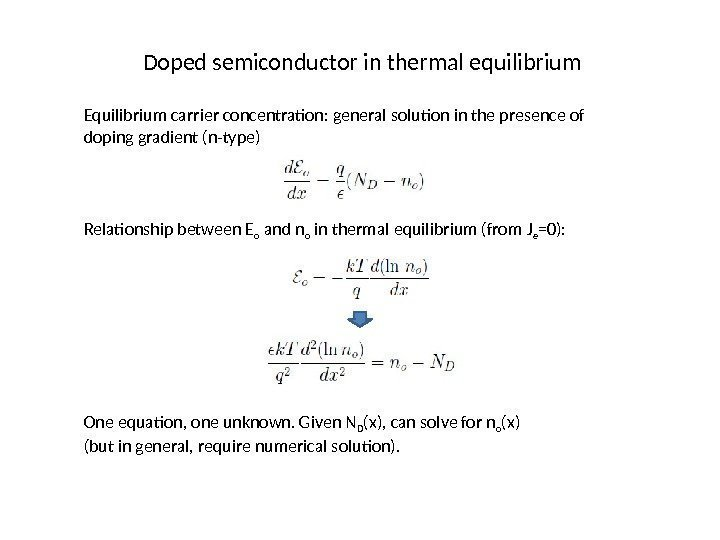Doped semiconductor in thermal equilibrium Equilibrium carrier concentration: general solution in the presence of doping gradient (n-type) Relationship between E o and n o in thermal equilibrium (from J e =0): One equation, one unknown. Given N D (x), can solve for n o (x) (but in general, require numerical solution).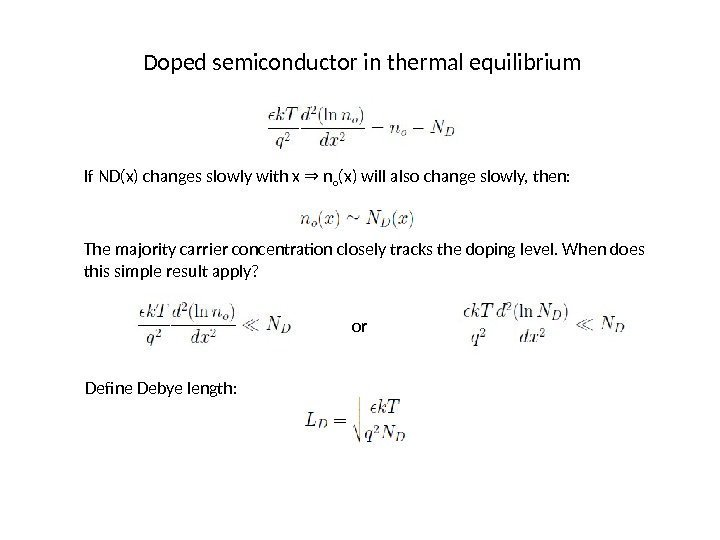Doped semiconductor in thermal equilibrium If ND(x) changes slowly with x n⇒ o (x) will also change slowly, then: The majority carrier concentration closely tracks the doping level. When does this simple result apply? or Define Debye length: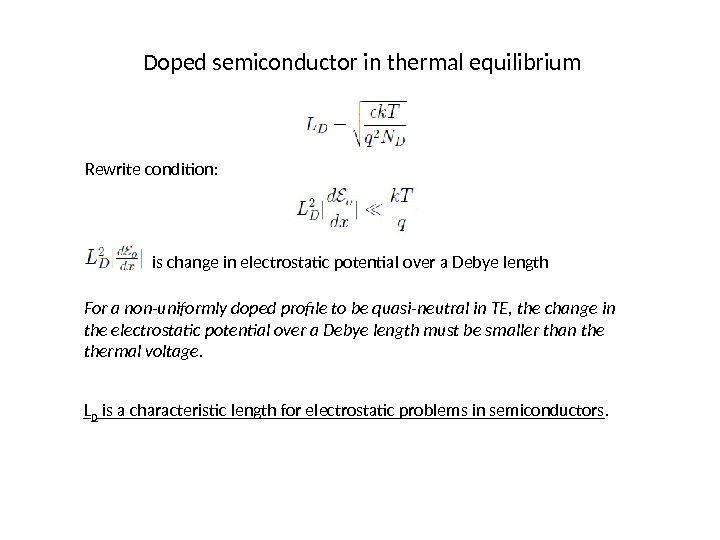Doped semiconductor in thermal equilibrium Rewrite condition: is change in electrostatic potential over a Debye length For a non-uniformly doped profile to be quasi-neutral in TE, the change in the electrostatic potential over a Debye length must be smaller than thermal voltage. L D is a characteristic length for electrostatic problems in semiconductors.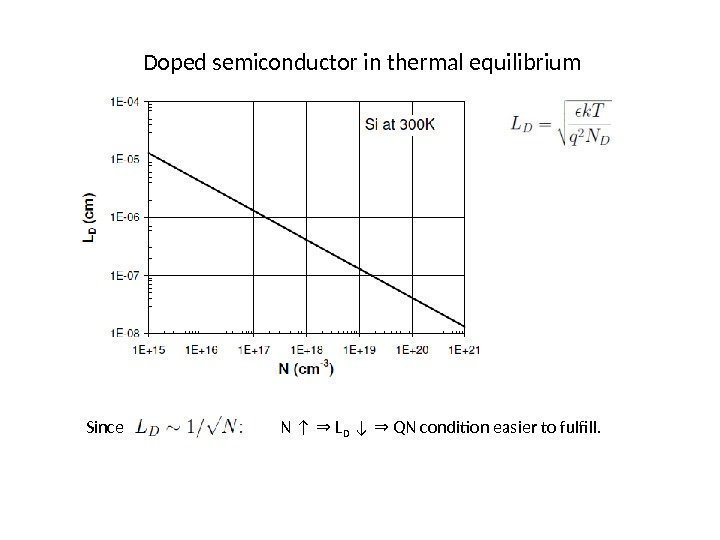Doped semiconductor in thermal equilibrium Since N ↑ L⇒ D ↓ QN condition easier to fulfill. ⇒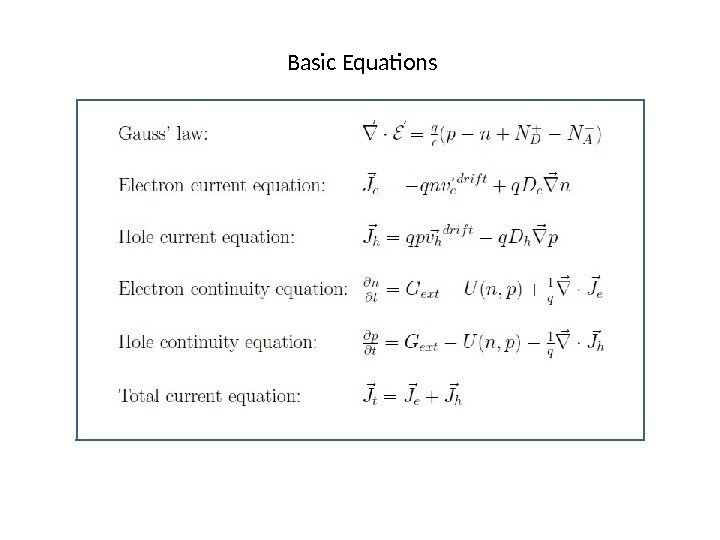Basic Equations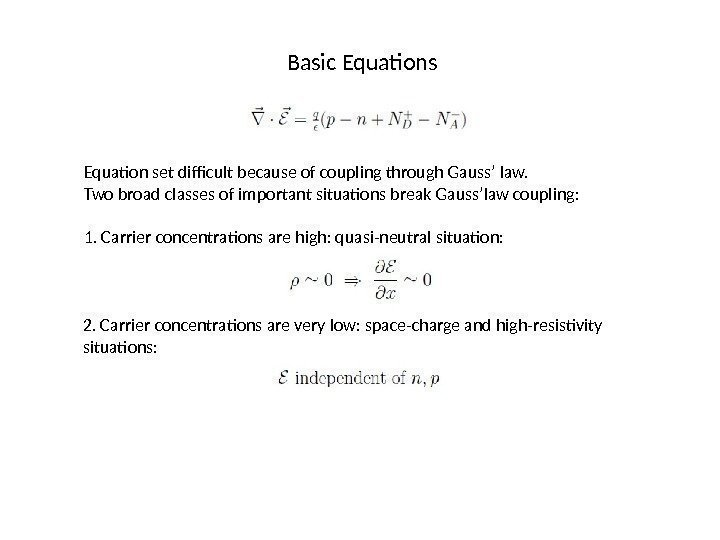Basic Equations Equation set difficult because of coupling through Gauss’ law. Two broad classes of important situations break Gauss’law coupling: 1. Carrier concentrations are high: quasi-neutral situation: 2. Carrier concentrations are very low: space-charge and high-resistivity situations: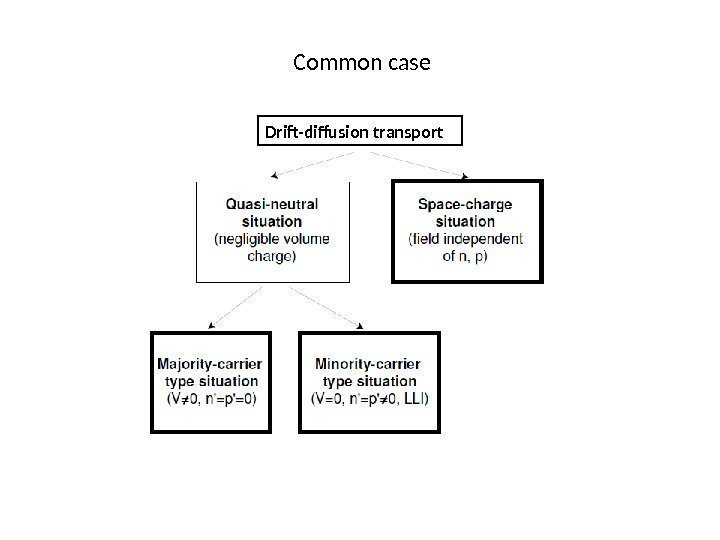Common case Drift-diffusion transport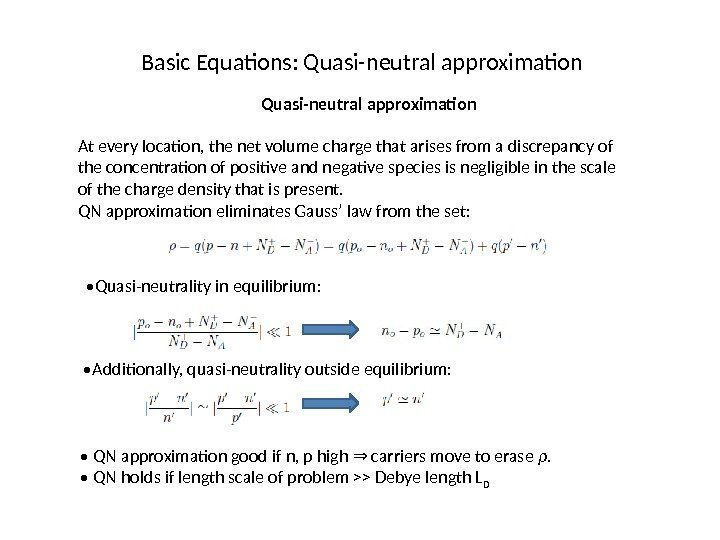Basic Equations: Quasi-neutral approximation At every location, the net volume charge that arises from a discrepancy of the concentration of positive and negative species is negligible in the scale of the charge density that is present. QN approximation eliminates Gauss’ law from the set: • Quasi-neutrality in equilibrium: • Additionally, quasi-neutrality outside equilibrium: • QN approximation good if n, p high carriers move to erase ⇒ ρ. • QN holds if length scale of problem >> Debye length L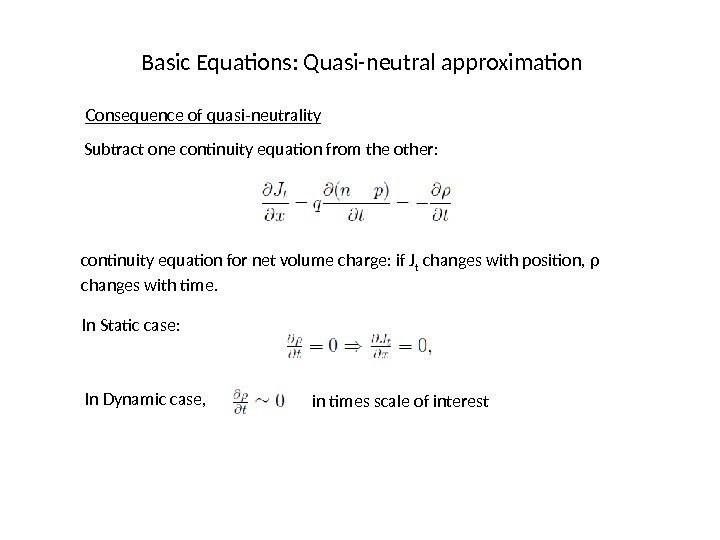Basic Equations: Quasi-neutral approximation Consequence of quasi-neutrality Subtract one continuity equation from the other: continuity equation for net volume charge: if J t changes with position, ρ changes with time. In Static case: In Dynamic case, in times scale of interest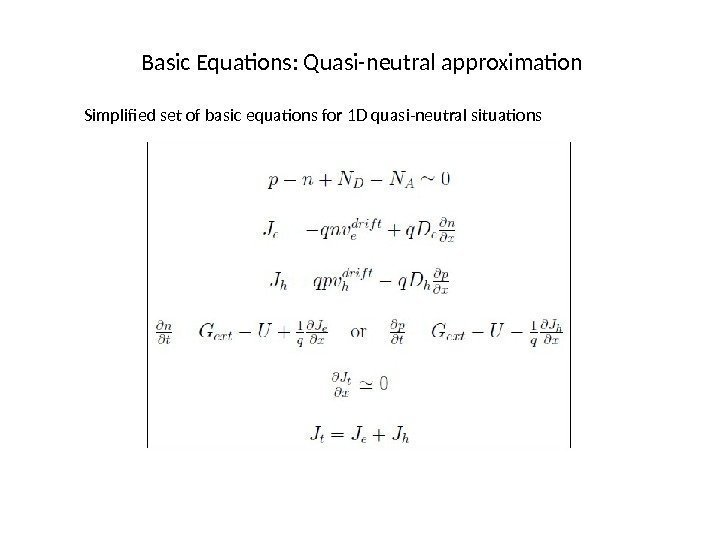Basic Equations: Quasi-neutral approximation Simplified set of basic equations for 1 D quasi-neutral situations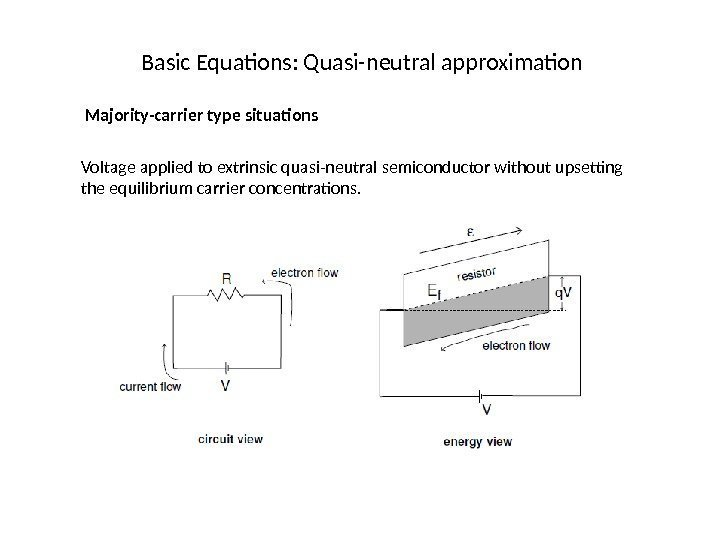Basic Equations: Quasi-neutral approximation Majority-carrier type situations Voltage applied to extrinsic quasi-neutral semiconductor without upsetting the equilibrium carrier concentrations.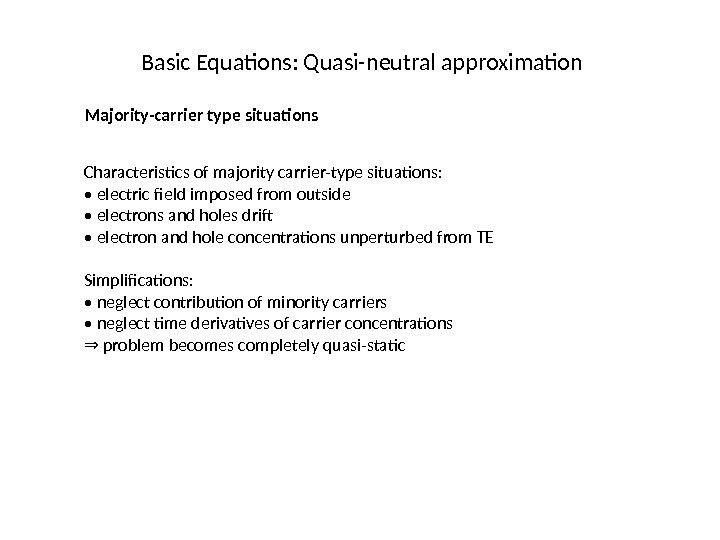Basic Equations: Quasi-neutral approximation Majority-carrier type situations Characteristics of majority carrier-type situations: • electric field imposed from outside • electrons and holes drif • electron and hole concentrations unperturbed from TE Simplifications: • neglect contribution of minority carriers • neglect time derivatives of carrier concentrations ⇒ problem becomes completely quasi-static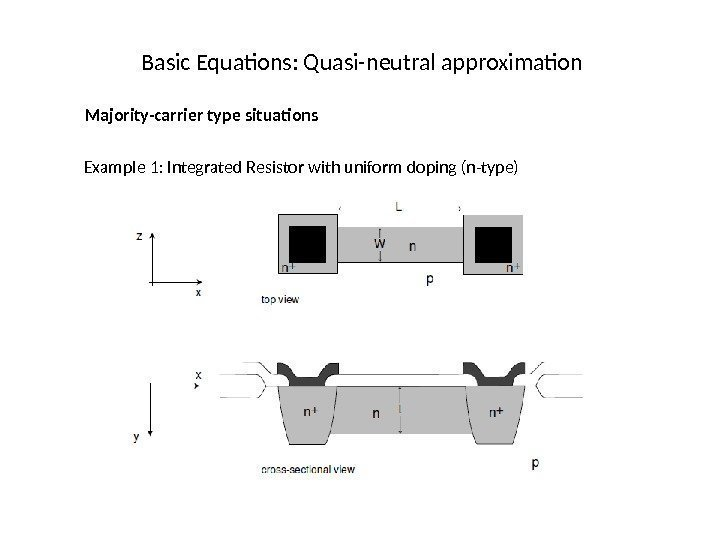Basic Equations: Quasi-neutral approximation Majority-carrier type situations Example 1: Integrated Resistor with uniform doping (n-type)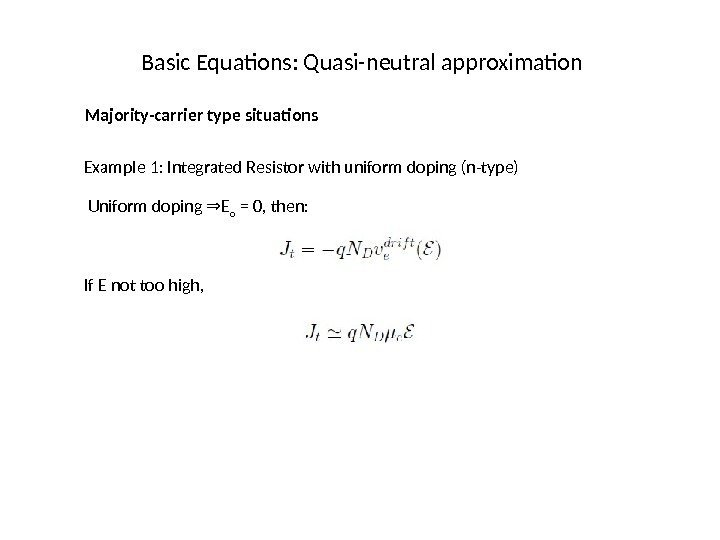Basic Equations: Quasi-neutral approximation Majority-carrier type situations Example 1: Integrated Resistor with uniform doping (n-type) Uniform doping E⇒ o = 0, then: If E not too high,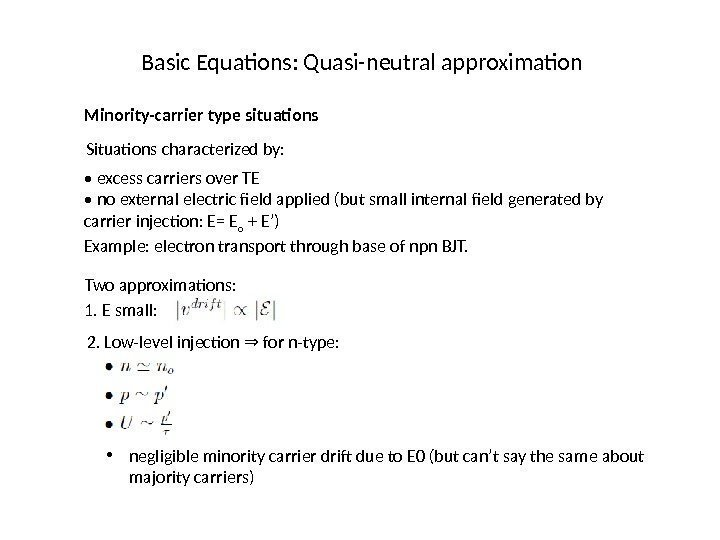Basic Equations: Quasi-neutral approximation Minority-carrier type situations Situations characterized by: • excess carriers over TE • no external electric field applied (but small internal field generated by carrier injection: E= E o + E’) Example: electron transport through base of npn BJT. Two approximations: 2. Low-level injection for n-type: ⇒ 1. E small: • negligible minority carrier drif due to E 0 (but can’t say the same about majority carriers)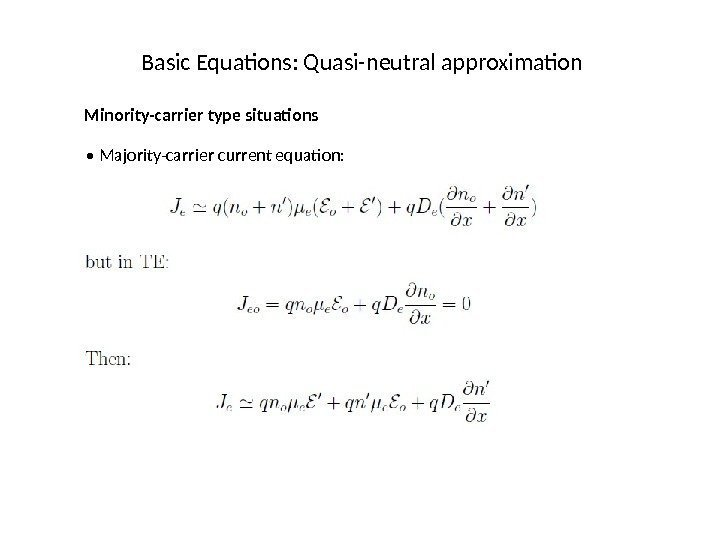Basic Equations: Quasi-neutral approximation Minority-carrier type situations • Majority-carrier current equation: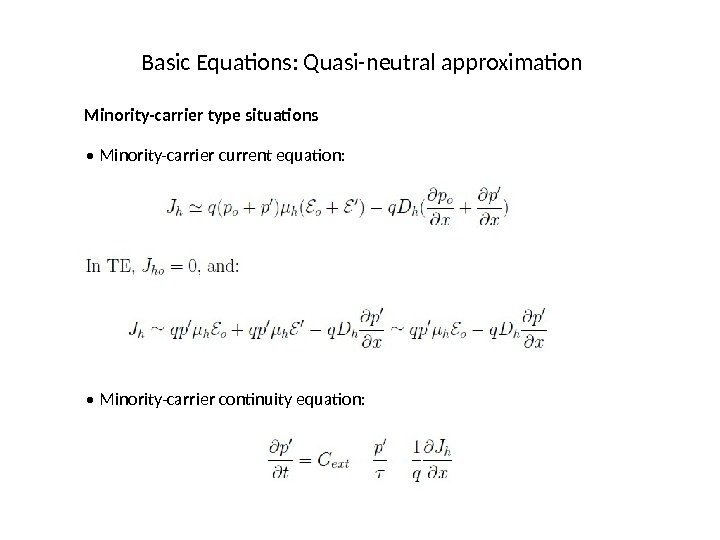Basic Equations: Quasi-neutral approximation Minority-carrier type situations • Minority-carrier current equation: • Minority-carrier continuity equation: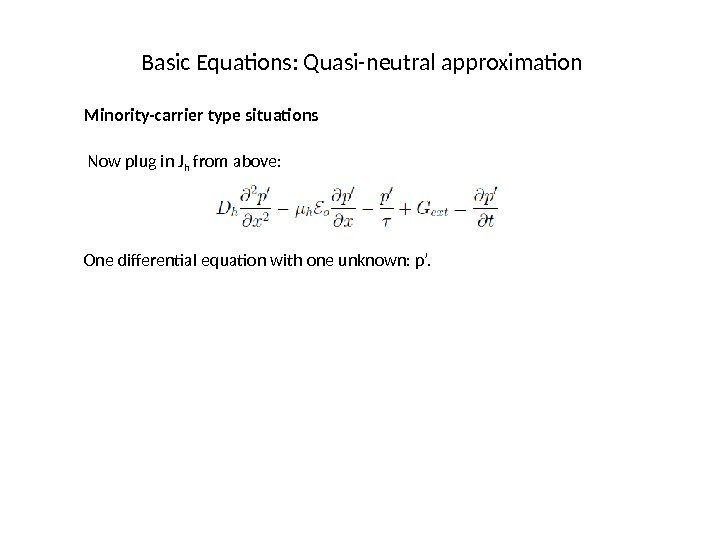Basic Equations: Quasi-neutral approximation Minority-carrier type situations Now plug in J h from above: One differential equation with one unknown: p’.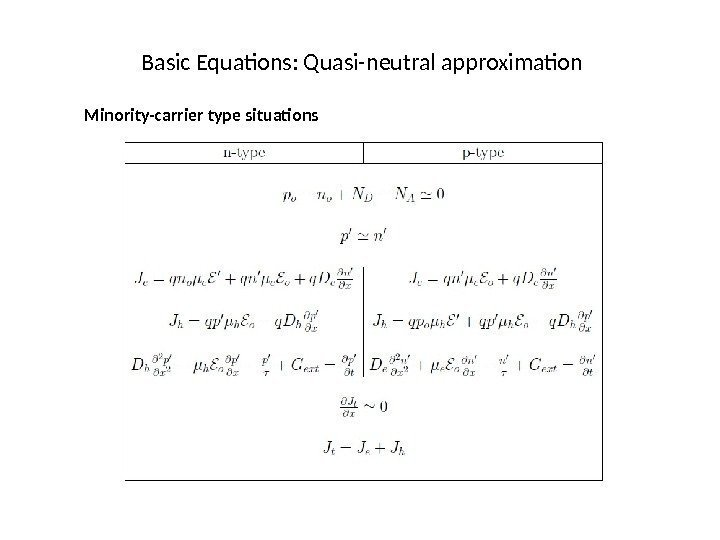Basic Equations: Quasi-neutral approximation Minority-carrier type situations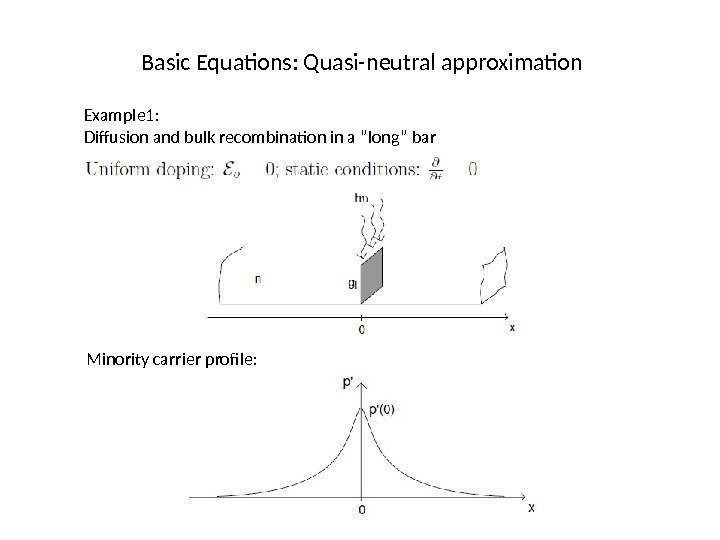Basic Equations: Quasi-neutral approximation Example 1: Diffusion and bulk recombination in a ”long” bar Minority carrier profile: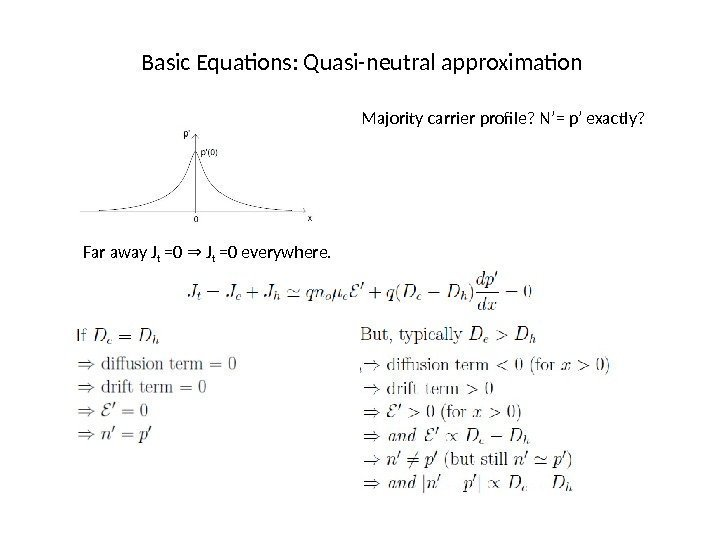Basic Equations: Quasi-neutral approximation Majority carrier profile? N’= p’ exactly? Far away J t =0 J⇒ t =0 everywhere.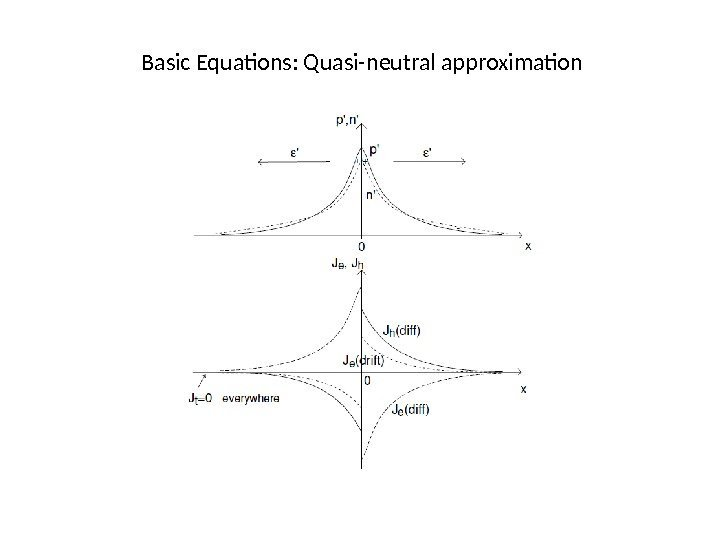Basic Equations: Quasi-neutral approximation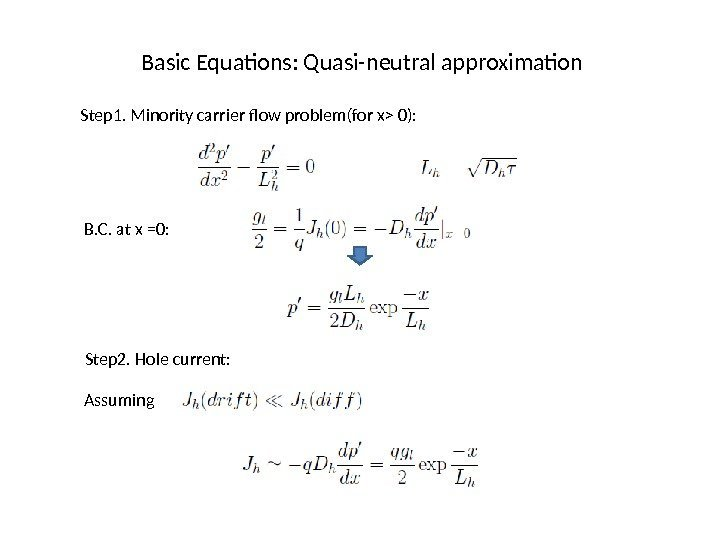Basic Equations: Quasi-neutral approximation Step 1. Minority carrier flow problem(for x> 0): B. C. at x =0: Step 2. Hole current: Assuming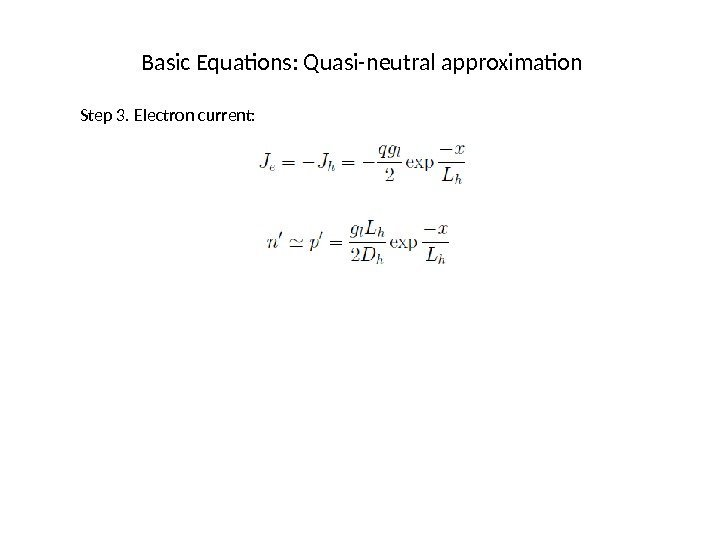Basic Equations: Quasi-neutral approximation Step 3. Electron current: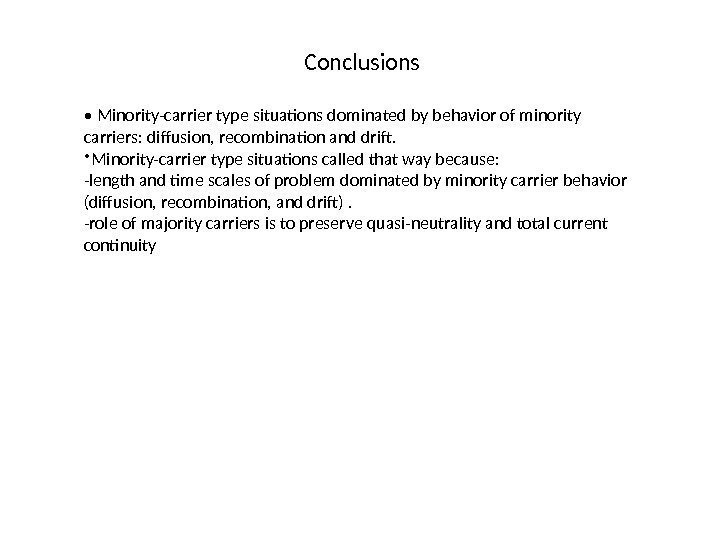Conclusions • Minority-carrier type situations dominated by behavior of minority carriers: diffusion, recombination and drif. • Minority-carrier type situations called that way because: -length and time scales of problem dominated by minority carrier behavior (diffusion, recombination, and drif). -role of majority carriers is to preserve quasi-neutrality and total current continuity